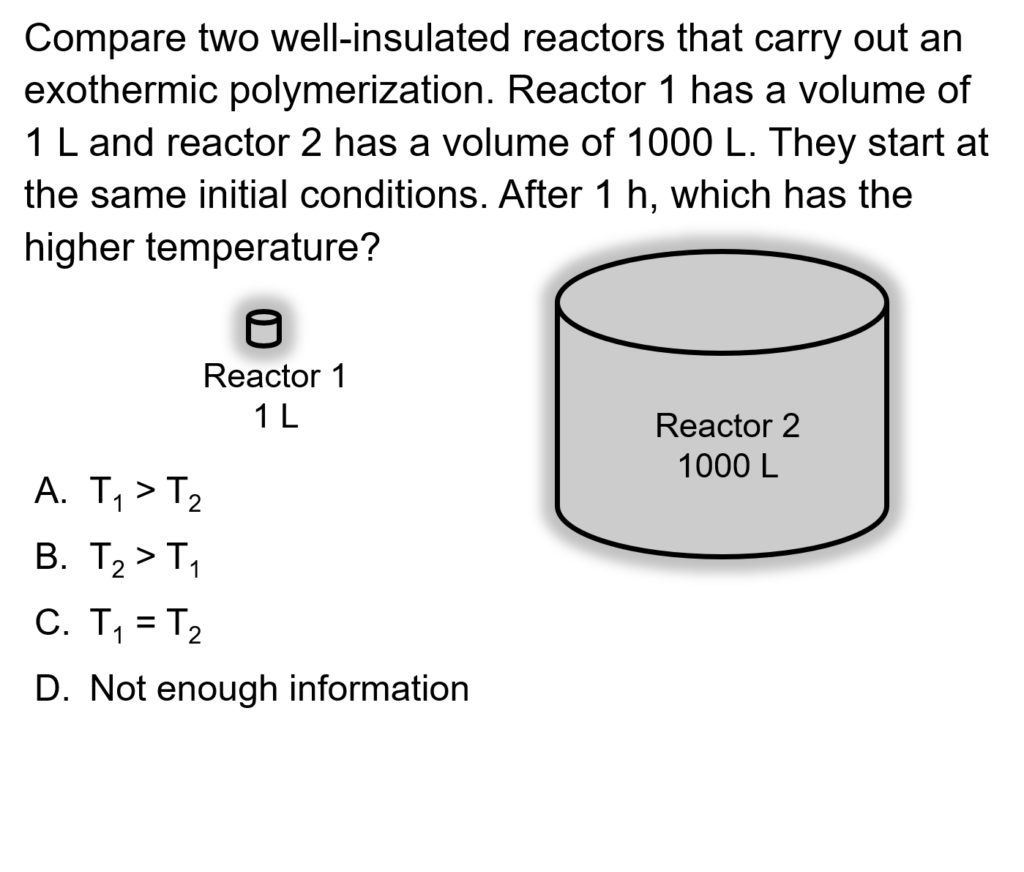LearnChemE

#### Energy Balances in Stirred Tank Reactors: ConcepTest and Example Problem

Try to answer this ConcepTest and solve this example problem before using this module. Studies show that trying to answer the questions before studying material improves learning and retention. By the end of this module, you should be able to answer these on your own. Answers will be given later in this module.##### Example Problem 1

The liquid-phase reaction A → B takes place in an adiabatic batch reactor. The initial temperature is 100°C. Initially, the reactor (100 L volume) contains only A (CA0 = 5 mol/L). The heat of reaction is -14.6 kJ/mol and is independent of temperature.

Heat capacity CPA = 83.6 J/(mol K)

Reaction rate rA = -kCA2.5     k = 2.5 x 10-3e(-760/T) (L/mol)1.5 s-1

What is the reactor temperature when 60% of A has reacted?  How long does it take to reach 60% conversion of A?